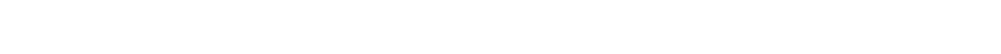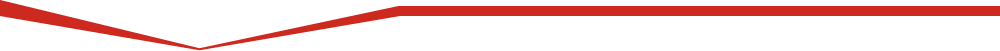Education Help with Education Education Resources UNAVCO Educator Packet Field Geodesy Learning Modules & Activities Majors-level undergraduate Introductory-level undergraduate Secondary- Grades 6-12 Data for Educators Tutorials & Handouts GPS Tutorials 3D Imaging Tutorials Geodesy Education Handouts & KMZs Lab Book Links

# Tutorial: The Geoid and Receiver Measurements

In this tutorial, you will learn about the difference between the reference ellipsoid and the geoid, and how these concepts come together to tell us the true elevation above sea level.

### Receiver Measurements and the Geoid

On the The Shape of Earth and Reference Ellipsoids page, we learned that GPS receivers use a smooth approximation of sea level called a reference ellipsoid to calculate elevation. The exact shape of sea level is complicated and can change place to place and from time to time due to the effects of ocean currents, tides, winds, and other factors. To avoid trying to store all of this variable information inside a GPS receiver, the simplified reference ellipsoid model of sea level is instead used by a GPS receiver. Because of this, the elevation reading on a GPS navigation system is actually the height above the reference ellipsoid and not the height above true sea level. Note that locations such as Death Valley, the Qattara Depression, and Dead Sea lie below sea level and so, the GPS receiver elevation reading is the distance below the reference ellipsoid.

### What Does the Real Sea Level Look Like: The Geoid Model

Let's review what we know so far:

1. Earth is approximately shaped like an ellipsoid.
2. A GPS receiver uses a smoothed map of sea level from which to approximate elevation.
3. The smoothed map is called a reference ellipsoid, and more specifically the WGS-84 Reference Ellipsoid.
4. Since the reference ellipsoid is only a model of the real-world situation, its surface does not match every point on the real sea level.

So, what does the real shape of sea level look like? In the The Shape of Earth and Reference Ellipsoids tutorial, we discussed that actual sea level is a rather complicated shape. Even after accounting for the effects of oceanographic and atmospheric factors such as winds, tides, and ocean currents, the actual surface of the ocean (what we call sea level) is still bumpy. It turns out that those bumps are a reflection of the mass distribution between that location and the center of the planet. Here’s why.

All objects have mass. Gravity is an attraction between two masses that causes them to accelerate towards one another. Without gravity, if you held a pencil in the air and let go, it would just float (like it does for astronauts in the space station). With gravity, if you hold the pencil and then let go it falls to the ground. More specifically, the pencil accelerates towards Earth and Earth accelerates towards the pencil. Now you might think, "I have never seen the ground move towards my pencil when I drop it!" That’s because Earth is SO MUCH BIGGER than everything on it so we can't detect the planet moving towards things when we drop them.

Below is a cartoon that depicts a pencil accelerating towards the center of Earth (big black arrow) and Earth accelerating towards the pencil (small black arrow). For more advanced students, the equation for gravitational force can be used to calculate the gravitational attraction between Earth (mass, m1 = 5.97 x 10^24 kg) and the pencil (mass, m2 = 0.001 kg). Try the calculation by assuming that the distance from the surface of Earth to its center is approximately 6,360,000 meters, and that your hand is roughly 1 meter above that surface. You can also calculate the acceleration due to gravity of Earth towards the pencil and the pencil towards Earth. Which acceleration is bigger? (Click here for the answer).We’ve just learned that the pencil and Earth accelerate towards each other because both have mass. Let’s now expand this concept of gravitational attraction and acceleration and apply it to answering the question of why the true shape of sea level is complex.

The ocean floor is far from being flat and featureless. In fact, underwater volcanoes, mountain chains, fractures, faults, trenches, and other features are found under our oceans. Furthermore, scientists have mapped rocks with different densities beneath the seafloor. Now, physics dictates that water has to come into equilibrium with the local gravitational attraction. As a result, the surface of the ocean molds itself into bumps and troughs to reflect these differing gravitational attractions. For example, a large volcano sitting on the seafloor tends to pull ocean water towards it, making the surface of the ocean pile up above it. For some large, dense volcanoes, the surface of the ocean is piled up to a height of a few meters above it! In contrast, an oceanic trench does not exert as much pull as do the surrounding regions so water moves away, creating a small trough in the ocean surface above the trench.

This bumpy ocean surface that is free of time-varying effects like tides and currents is called the geoid, or reference geoid. In continental areas, the undulatory surface of the reference geoid can be estimated as the level that would be taken by the surface of the sea in a set of criss-crossing canals stretching across the land from coast to coast. Just like the ocean floor, land surfaces have mountains, valleys, and other topographic features that affect the local gravity field and thus, the reference geoid. The complicated nature of the reference geoid explains why a simplified smooth reference ellipsoid is instead used in GPS receivers!

Below is an image of what the real sea level looks like for the entire world. The purple and blue spots are depressions in the sea level, while the red areas are high points. This image is a picture of the reference geoid. There are, in fact, different reference geoids for different areas of the world, depending upon which specific reference level is desired. The global version shown here is called the WGS-84/EGM96 reference geoid. It is the current best model we have for approximating sea level (the actual surface of the ocean).Image source is National Geodetic Survey's webpage at http://www.ngs.noaa.gov/images/ngs/jpeg-geo/ww15mgh.jpg

### Putting it All Together

Topographic surface elevations (the height of the land) are usually reported as the distance in meters or feet above or below sea level, which means the distance above or below the reference geoid. But, our GPS receiver measures the height of the receiver above the smooth reference ellipsoid. So, how do we calculate the GPS receiver height above the reference geoid?We know the elevation of the GPS receiver above the reference ellipsoid (this height is often called the ellipsoidal height). And, from a sea level map like the WGS-84/EGM96 Geoid we know the height of the reference geoid at our latitude and longitude above the reference ellipsoid - you can find this value using UNAVCO's Geoid Height Calculator. So, the topographic height of the receiver above sea-level is simply the difference between these two values:

 Topographic Height = Ellipsoidal Height - Geoid Height

In summary, height above sea level is equal to the difference between the height above the reference ellipsoid MODEL and the height of the reference geoid at that point on the Earth.

Example
1. Sally took 15 readings of her position with a GPS receiver and then calculated the average of these readings, which she wrote down.

Latitude = 40 degrees 2.08 minutes North
Longitude = 104 degrees 14.25 minutes West
Elevation = 1602 meters (ellipsoidal height)
2. Next Sally visited our on-line geoid height calculation website. She entered her latitude and longitude where indicated and got a geoid height of -15.9 meters.

Geoid Height = -15.9 meters
3. Finally, Sally used these numbers to calculate her topographic height.

 Topographic Height = Ellipsoidal Height - Geoid Height Topographic Height = 1602 meters - -15.9 meters Topographic Height = 1617.9 meters